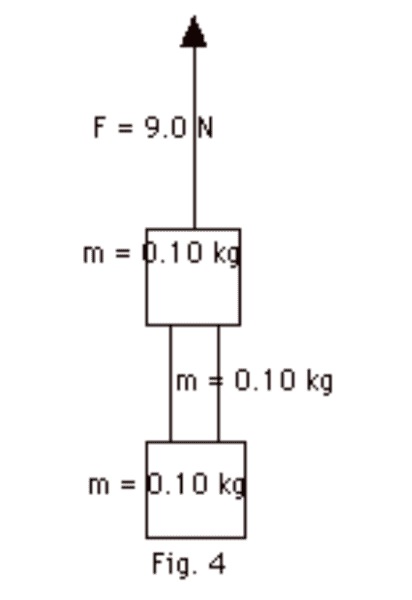# Tension on a massive rope

## Homework Statement

A system, consisting of a wide rope of mass 0.10 kg between two blocks each of mass 0.10 kg, is lifted by an applied force F = 9.0 N (Fig. 4 below). (a) Find the acceleration of the system. Find the tension at (b) the top of the rope, and (c) the bottom of one-fifth of the rope. Take g = 10 m/s2.Fnet=ma

## The Attempt at a Solution

a) Fnet=ma
F- 3mg = ma
9- 3(0.1)(10) = 0.1a
6=0.1 a
a= 60 m/s^2

b) T- 2mg = ma
T-2(0.10)(10) = 0.10(60) (it's right to use the mass of rope by acceleration right?)
T-2=66
Ttop= 68N
is it right?

c) T- (4/5x m)- 10xm= (4/5)m x a
T- 0.08(10) - (0.10)(10)= 0.08(60) is this equation right
i don't if i got (the bottom of one-fifth of the rope) correctly or not. i considered the tension to equal to that part of the rope below 1/5 from the mass so that m of rope now is 4/5m
and also i have the lower mass (m). so is it right??

#### Attachments

PeroK
Homework Helper
Gold Member
2021 Award
a) Fnet=ma
F- 3mg = ma
9- 3(0.1)(10) = 0.1a
6=0.1 a
a= 60 m/s^2

Doesn't that seem a bit high to you?

b) T- 2mg = ma
T-2(0.10)(10) = 0.10(60) (it's right to use the mass of rope by acceleration right?)
T-2=66
Ttop= 68N
is it right?

You have a ##9N## force and a tension of ##68N## Does that makes sense?

•SakuRERE
Doesn't that seem a bit high to you?

You have a ##9N## force and a tension of ##68N## Does that makes sense?
it does, that's why I am asking here. i get number that are too big

PeroK
Homework Helper
Gold Member
2021 Award
it does, that's why I am asking here. i get number that are too big

What is the mass of your system?

What is the net external force on your system?

What is the acceleration of your system?

What is the mass of your system?
the total mass is 3(0.10) the two blocks and the rope
fnet is 9N
the acceleration... do you mean it should be F- 3(mg)=3ma????

PeroK
Homework Helper
Gold Member
2021 Award
the total mass is 3(0.10) the two blocks and the rope

If you mean: The total mass is ##0.3kg##, then yes.

fnet is 9N

the acceleration... do you mean it should be F- 3(mg)=3ma????

I didn't mean anything. I just asked a question!

the gravity force is down and each mass including the rope will have a weight downward.
I didn't mean anything. I just asked a question!
Oh yeah, i am just trying to reach the solution fast :)

PeroK
Homework Helper
Gold Member
2021 Award
the gravity force is down and each mass including the rope will have a weight downward.

Exactly, so you need to take gravity into account when calculating the external force on your system.

i JUst checked again my solution and i must have put 3m in a)
F-3mg= (3M)a
right? so a=20

and regarding the b) it would be
T-2mg=2ma
right?

and for c)
it would be
T-(1/5x0.10)g - 0.10g = (1/5x0.10+ 0.10) (20)
right?

PeroK
Homework Helper
Gold Member
2021 Award
i JUst checked again my solution and i must have put 3m in a)
F-3mg= (3M)a
right? so a=20

20 what? Units are important.

and regarding the b) it would be
T-2mg=2ma
right?

That's the idea. It's called Newton's 2nd Law!

•SakuRERE
20 what? Units are important.
a= 20 m/s^2

so it's right and i am done with it right?

PeroK
•